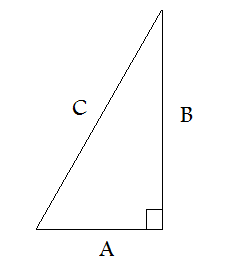# Absurdity

Jan 06 2010 - Jun 08 2014

## Pythagorean Theorem

### Explanation:A² + B² = C². This is true in a right-angled triangle, a right angled triangle is a triangle where one of the interior angles is equal to 90°. A and B are both sides of the right-angle, whereas C is the side directly opposite of the 90° angle, the hypotenuse. A and B are interchangeable, C is not. To use this equation you must already know two of the sides in the triangle. Using this with trigonometry we can determine all the angles and sides of a triangle with minimal information, all we would need is 1 side and 1 angle other than the right-angle.

#### Try It Out:

In the example below simply fill out two of the three blanks and the script will calculate and display the remaining side, to three decimal points, with all the work inbetween.

A² + B² = C²

+ =